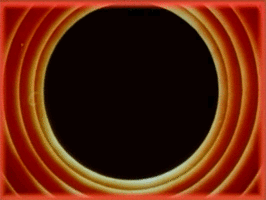Averaging RGB Colors the Right Way

This is a quick tip for those who find themselves needing to average multiple RGB colors for some reason.

The typical approach to averaging RGB colors is to add up all the red, green, and blue values, and divide each by the number of pixels to get the components of the final color.

There’s a better way!

Instead of summing up the components of the RGB color, sum their squares instead. Then when returning the average color, for each color component, find the mean of the sum (of squares) and return its square root.

Here’s an image that has been recreated using a million randomly-placed circles of varying radii, each filled with the average color of all pixels in that region in a reference photo. You can see the difference between the simple approach (no squaring of RGB components) and the squared approach.The difference is subtle, but you’ll notice that the colors are lighter in the squared one, and in fact are closer in brightness to the reference image. h This is particularly evident in the brighter parts (e.g., observe the bright-yellow part at the back of the boat).To understand why this works better, here’s a video explaining it in much more detail than I ever could.

Averaging RGB Colors in Processing

I always like to provide code as it helps to really drill down into the details when in doubt. With that in mind, here’s an example of a function in Processing that averages the pixels in a circular region of an image using the “simple” approach:

/*
* Averages the pixels in a given image (img) within a circular
* region centered at (x, y) with a radius of 'radius' pixels.
* This function uses the "simple" approach to average RGB
* colors which simply returns the mean of the red, green, and
* blue components.
*/
color getAverageRGBCircle(PImage img, int x, int y, int radius) {
float r = 0;
float g = 0;
float b = 0;
int num = 0;
/* Iterate through a bounding box in which the circle lies */
for (int i = x - radius; i < x + radius; i++) {
for (int j = y - radius; j < y + radius; j++) {
/* If the pixel is outside the canvas, skip it */
if (i < 0 || i >= width || j < 0 || j >= height)
continue;

/* If the pixel is outside the circle, skip it */
if (dist(x, y, i, j) > r)
continue;

/* Get the color from the image, add to a running sum */
color c = img.get(i, j);
r += red(c);
g += green(c);
b += blue(c);
num++;
}
}
/* Return the mean of the R, G, and B components */
return color(r/num, g/num, b/num);
}

Instead of using the function above, just make the following changes to it.

color getAverageRGBCircle(PImage img, int x, int y, int radius) {
...
for (int i = x - radius; i < x + radius; i++) {
for (int j = y - radius; j < y + radius; j++) {
...
/* Sum the squares of components instead */
r += red(c) * red(c);
g += green(c) * green(c);
b += blue(c) * blue(c);
...
}
}
/* Return the sqrt of the mean of squared R, G, and B sums */
return color(sqrt(r/num), sqrt(g/num), sqrt(b/num));
}

The only difference is in the way we add up the components of the RGB color and how we return them. Hope this helped!